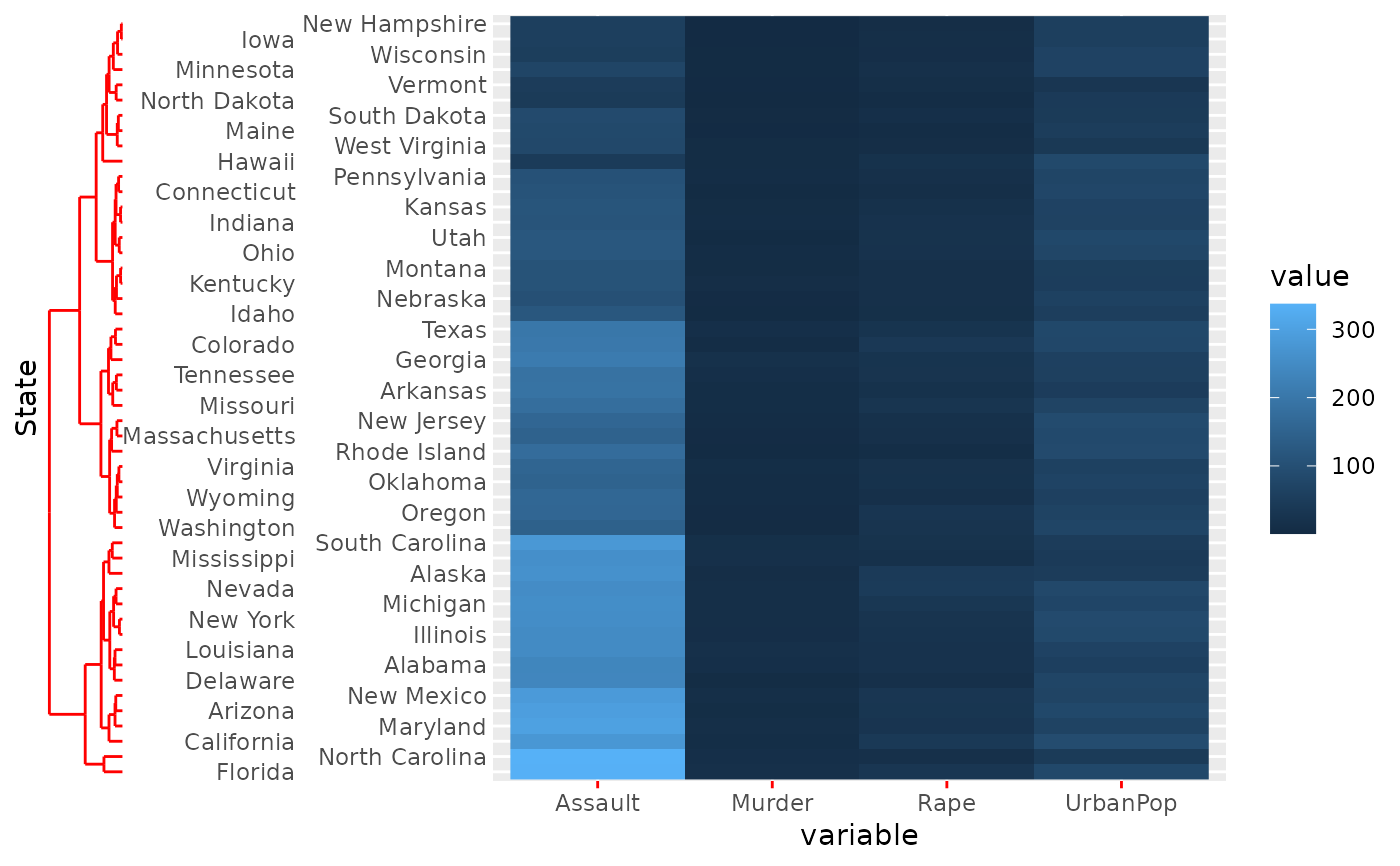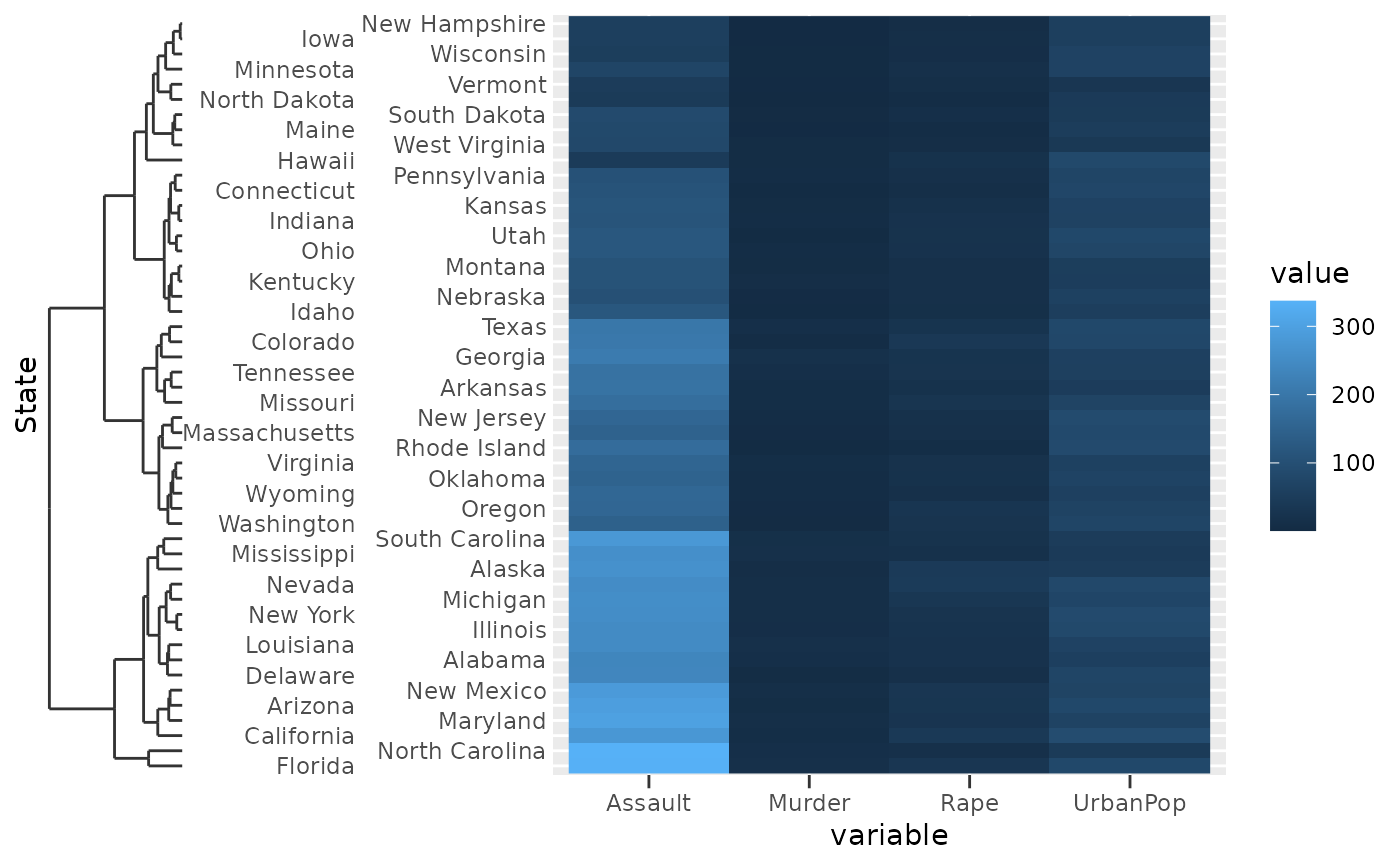Visual representation of a discrete variable with hierarchical relationships between members, like those detailed in scale_(x|y)_dendrogram)().

## Usage

guide_dendro(
title = waiver(),
check.overlap = FALSE,
n.dodge = 1,
order = 0,
position = waiver(),
label = TRUE,
trunc_lower = NULL,
trunc_upper = NULL,
colour = NULL,
color = NULL,
dendro = waiver()
)

## Arguments

title

A character string or expression indicating a title of guide. If NULL, the title is not shown. By default (waiver()), the name of the scale object or the name specified in labs() is used for the title.

check.overlap

silently remove overlapping labels, (recursively) prioritizing the first, last, and middle labels.

n.dodge

The number of rows (for vertical axes) or columns (for horizontal axes) that should be used to render the labels. This is useful for displaying labels that would otherwise overlap.

order

Used to determine the order of the guides (left-to-right, top-to-bottom), if more than one guide must be drawn at the same location.

position

Where this guide should be drawn: one of top, bottom, left, or right.

label

A logical(1). If TRUE, labels are drawn at the dendrogram leaves. If FALSE, labels are not drawn.

trunc_lower, trunc_upper

The lower and upper range of the truncated axis:

• NULL to not perform any truncation.

• A function that takes the break positions as input and returns the lower or upper boundary. Note that also for discrete scales, positions are the mapped positions as numeric.

• A numeric value in data units for the lower and upper boundaries.

• A unit object.

colour, color

A character(1) with a valid colour for colouring the axis text, axis ticks and axis line. Overrules the colour assigned by the theme.

dendro

Relevant plotting data for a dendrogram such as those returned by ggdendro::dendro_data().

## Value

A dendroguide class object.

## Details

The dendrogram guide inherits graphical elements from the axis.ticks theme element. However, the size of the dendrogram is set to 10 times the axis.ticks.length theme element.

## Examples

clust <- hclust(dist(USArrests), "ave")

# Melting USArrests
df <- data.frame(
State = rownames(USArrests)[row(USArrests)],
variable = colnames(USArrests)[col(USArrests)],
value = unname(do.call(c, USArrests))
)

# The guide function can be used to customise the axis
g <- ggplot(df, aes(variable, State, fill = value)) +
geom_raster() +
scale_y_dendrogram(hclust = clust,
guide = guide_dendro(n.dodge = 2))

# The looks of the dendrogram are controlled through ticks
g + theme(axis.ticks = element_line(colour = "red"))# The size of the dendrogram is controlled through tick size * 10
g + theme(axis.ticks.length = unit(5, "pt"))